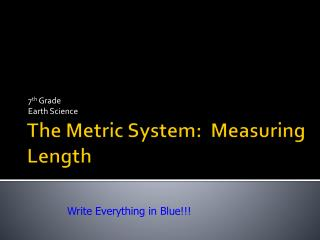DownloadDownload PresentationThe Metric System: Measuring Length

# The Metric System: Measuring Length

Télécharger la présentation## The Metric System: Measuring Length

- - - - - - - - - - - - - - - - - - - - - - - - - - - E N D - - - - - - - - - - - - - - - - - - - - - - - - - - -
##### Presentation Transcript

1. 7th Grade Earth Science The Metric System: Measuring Length Write Everything in Blue!!!

2. History • The metric system evolved over the years and in the 1960’s was named the International System of Measurement (SI for short). • Scientific standards were set with the gram, meter, kelvin, second, ampere, candela, & in 1971 – the mole

3. Advantages of Metric System • No numbers to memorize • Only 30 individual units • Easy to say and write • No fractions only decimals • World standard • Converts easily by multiples of 10.

4. Units of Measurement • Prefixes and Multiplier: • Kilo = 1000 • Hecto = 100 • Deca = 10 • Meter/Gram/Liter= 1 • Deci = .1 • Centi = .01 • Milli = .001 • Examples: • Kilometer • Hectometer • Decameter • Meter • Decimeter • Centimeter • Millimeter

5. Length: • The standard unit of length in the metric system is the meter. Other units of length and their equivalents in meters are as follows: 1 millimeter = 0.001 meter1 centimeter = 0.01 meter1 decimeter = 0.1 meter1 decameter = 10 meters 1 hectometer = 100 meters 1 kilometer = 1000 meters

6. Metric Length • If you need to measure length, you use the “meter” • If you need to measure longer lengths, you can use the kilometer • Lets say you live 10 miles from school • 10 miles = 16093 meters • 16093 is a large number, so you can move the decimal 3 spots to make it 16.1 kilometers

7. Abbreviations: • We abbreviate these lengths as follows:1 millimeter = 1 mm1 centimeter = 1 cm1 meter = 1 m1 decimeter = 1 dm1 kilometer = 1 km

8. Examples: • For reference, 1 meter is a little longer than 1 yard or 3 feet. It is about half the height of a very tall adult. A centimeter is nearly the diameter of a dime, a little less than half an inch. A millimeter is about the thickness of a dime.

9. Who Does Not Use It? • The only countries not to use the Metric System are the U.S., Myanmar, and Liberia

10. English to Metric Conversions • 39,370 inches = 1 kilometer • 3280.8 feet = 1 kilometer • .62 miles = 1 kilometer • 39 inches = 1 meter • 3.28 feet = 1 meter • 1 inch = 254 mm • 1 inch = 2.54 cm • 1 foot = 30.48 cm • 1 yard = 91.44 cm • 1 yard = .914 m • 1 mile = 1609.3 m • 1 mile = 1.609 km You do not need to know these. They are just for you to get an idea of how these systems compare.

11. Comparisons

12. Metric Measurements The 3 main measurements are the gram (weight), meter (length), & liter (volume)

13. Metric Prefixes • These prefixes are based on powers of 10. • What does this mean? • From each prefix every “step” is either: • 10 times larger or • 10 times smaller • For example • Centimeters are 10 times larger than millimeters • 1 centimeter = 10 millimeters

14. Metric Prefixes • For each “step” to right, you are multiplying by 10 • For example, let’s go from a base unit to centi 1 liter = 10 deciliters = 100 centiliters 2 grams = 20 decigrams = 200 centigrams

15. Summary • Summary • Base units in the metric system are meter, liter, gram • Metric system is based on powers of 10 • For conversions within the metric system, each “step” is 1 decimal place to the right or left